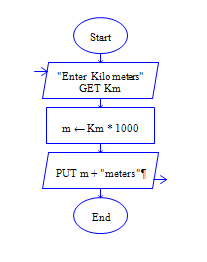# C Program to convert Kilo Meters to Meters

Get Kilometer km from user and find meter m

Sample Input 1:

56

Sample Output 1:

56000

#### Flow Chart DesignStrongly recommended to Solve it on your own, Don't directly go to the solution given below.

#include<stdio.h> int main() { //write your code here }

#### Program or Solution

``` #include <stdio.h> int main(void) { int m; float km; printf("enter kilometers:"); scanf("%f",&km); m=km*1000; printf("Meters: %d",m); return 0; } ```

#### Program Explanation

Get kilometer km as input (using scanf statement) (since kilometer may be in fractional values like 1.2 and 5.2, it is declared & read as float.)

Calculate meter m by multipllying kilometer km with 1000.

(m=km*1000, 1000 meter is equal to 1 kilometer) print meter m.

(using printf statement)